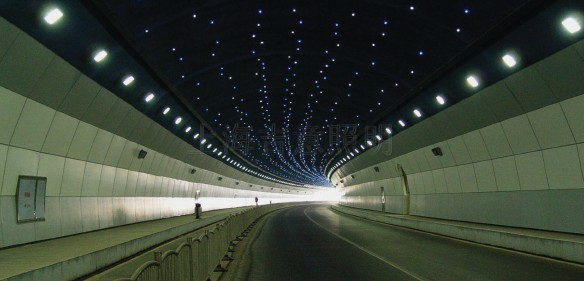# The wiring method and load calculation of led display power supplyHow to connect led display power line?
The power cord between the led display unit plates is in parallel. A power supply usually has 3 sets of 5V output. Can Be 10 to 12 P10 plates. With more than one power source, the 220 input from the power source is in parallel. The power cord of the Control Card is best printed directly from the power source, not through the unit plate.

Here are two main ways to connect:

"T" type connection.

Brief introduction:

Power supply a set of 2.5 was output line with four P10 module, top-down Zhang Mo group 2 to the power supply output line terminal, module 1 and module 3, modules in parallel state 4, current and shunt.

"One" font connection.

Brief introduction:

A 2.5mm2 output line of the power supply has 4 P10 modules, and the power output line is connected to the first module of the top end. The current is supplied from top to bottom with four modules.

The single P10 module works with A maximum current of 4.2A. According to T - type connection. The connecting line of the power supply connecting line of the 1mm2 module is the maximum, and the connecting line of module 2 to module 3 is responsible for the power supply of module 3 and module 4. The current is 4.2*2=8.4A, which is in the normal range of the cross sectional area of 1mm2.

The maximum working current of the single module is 4.2A. According to 1 type connection with load, the cross-sectional area of 1 was maximize module connecting current for module 1 and module 2 module connecting line, the line for module 2, 3, 4 power supply module, module by current 4.2 * 3 = 12.6 A. The carrying range of the 1mm2 wire has exceeded the cross-sectional area, which can lead to the heating of the wire, softening of the insulation and even the fire.

LED display technology.

Load calculation of switching power supply.

How many boards can a power supply take?

LED display power is generally 5V. When the board is positive and negative, there will be no problem, but also be careful not to let the positive negative short circuit. The power base on the board is connected to 5V and GND, and the positive and negative terminals cannot be connected. The power supply is only divided into VCC(5V) and GND.

Calculation method of power number of LED display screen:

(the power supply is 30A and 40A; The monochrome is a power supply of 8 unit plates with a 40A, and the double color is a power supply of 6 units. If the full color panel is good for the maximum power at full brightness

A) a power to take a couple of unit plate number = power supply voltage * power of current/cell plate of horizontal pixel points/cell plate vertical pixel points / 0.1/2 such as: half outdoor P10:5 v40a power can bring: 40/5 * (32 * 16 * 0.1/0.5) = 7.8 take big eight;

B) according to the number of screen's total power and the required power = average total power/power of a power supply (power supply voltage * power supply current), for example: a bar screen long with 12 P10 module, high with three P10 module: a total of 36 module So the required power number = 32 * 16 * 0.1 * 36 * 40 = 4.6/0.5/5 (five power);

C) power calculation method of LED display screen: the formula of power is P=UI; P stands for power, U stands for voltage, I stands for current, and usually we use 5V for the power supply, and the power supply is 30A and 40A. The monochrome is a power supply of 8 unit plates with a 40A, and the double color is a power supply of 6 units. The power of the outdoor screen is clearly defined in terms of "product parameters" on the website.

Here is an example: a unit will do 9 square meters of indoor 5.0 dual-color electronic screen, calculate the maximum required power. First, we need to figure out the number of power supply of 40A =9(0.244*0.488)/6=12.5=13 power supply (to be an integer, to be a great standard), so it's very simple, the maximum power P=13 *40A*5V=2600W. Single lamp power = a lamp power 5 v * 20 ma = 0.1 WLED display unit panel power = * resolution power of the single lamp (horizontal pixel points * vertical pixel points) / 2, the resolution of the screen body maximum power = screen body * per lamp number * 0.1 screen resolution of the resolution of the average power = screen * each lamp number * 0.1/2 resolution, the resolution of the screen body of the actual power = screen body * every count * 0.1 / scanning resolution lamp (4, 2, 16, 8, static).

Please feel free to give your inquiry in the form below. We will reply you in 24 hours.
Message